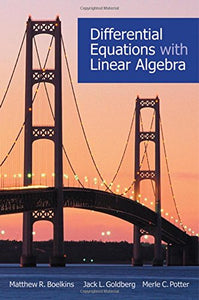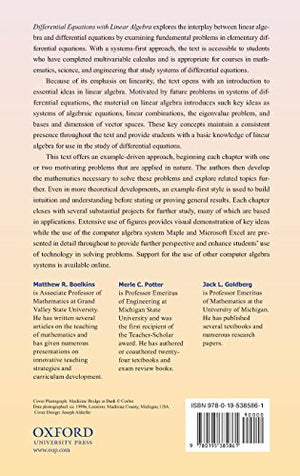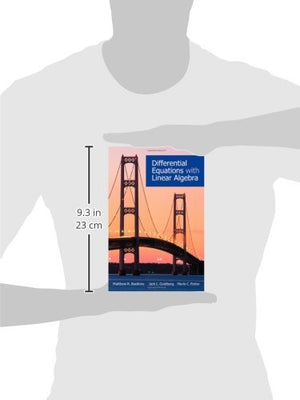Differential Equations with Linear Algebra

• Publish Date: 2009-11-05
• Binding: Hardcover
• Author: Matthew R. Boelkins;Jack L. Goldberg;Merle C. Potter
Regular price Sale price \$81.31

Linearity plays a critical role in the study of elementary differential equations; linear differential equations, especially systems thereof, demonstrate a fundamental application of linear algebra. In Differential Equations with Linear Algebra, we explore this interplay between linear algebra and differential equations and examine introductory and important ideas in each, usually through the lens of important problems that involve differential equations. Written at a sophomore level, the text is accessible to students who have completed multivariable calculus. With a systems-first approach, the book is appropriate for courses for majors in mathematics, science, and engineering that study systems of differential equations.

Because of its emphasis on linearity, the text opens with a full chapter devoted to essential ideas in linear algebra. Motivated by future problems in systems of differential equations, the chapter on linear algebra introduces such key ideas as systems of algebraic equations, linear combinations, the eigenvalue problem, and bases and dimension of vector spaces. This chapter enables students to quickly learn enough linear algebra to appreciate the structure of solutions to linear differential equations and systems thereof in subsequent study and to apply these ideas regularly.

The book offers an example-driven approach, beginning each chapter with one or two motivating problems that are applied in nature. The following chapter develops the mathematics necessary to solve these problems and explores related topics further. Even in more theoretical developments, we use an example-first style to build intuition and understanding before stating or proving general results. Over 100 figures provide visual demonstration of key ideas; the use of the computer algebra system Maple and Microsoft Excel are presented in detail throughout to provide further perspective and support students' use of technology in solving problems. Each chapter closes with several substantial projects for further study, many of which are based in applications.

Errata sheet available at: www.oup.com/us/companion.websites/9780195385861/pdf/errata.pdf